## Week 3 Coding Lecture 3: Computational complexity

We again include a recap of the theory, but we will skip to the coding, and for the theoretical details please see the theory lecture.
It is generally not enough to be able to solve a problem numerically; we also usually need our solution to be efficient. To figure out how efficient our method is, we need an estimate of how much work we actually have to do to solve a system. The usual approach with numerical methods is to give a rough estimate of how the work changes with the size of our problem. "Problem size" is a somewhat amorphous concept and it can often be difficult to come up with a good definition. In our case, we are interested in systems of N equations with N variables, so we will use N as a measure of the size of our problem. So, for instance, the first example we looked at in the last lecture had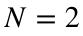and the second example had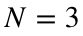. It is very common to have to solve systems with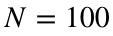or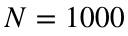, and there are many fields where problems may be much larger than that.
Detailed estimates of speed are certainly important, but they quickly become very difficult. One of the reasons for this difficulty is that the fundamental operations that your computer executes when you type a line of code can vary widely from one version of MATLAB to another, and even from one computer to another. Since we can't possibly go into all of these details, our estimates will be quite rough. This means that we are free to ignore what might seem like substantial details, since these details will be affected by many other issues that we can't measure. (For instance, we will throw away all constant factors whenever convenient, so we would treat N steps the same as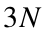steps or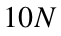steps.) Fortunately, our rough estimates will still prove very useful, and most mathematicians, computer scientists and programmers never have to worry about better estimates than these.
Before we can start, we need to know what fundamental steps (usually called instructions or operations) our computer can execute. As mentioned above, this varies from computer to computer, but we will assume that our processor can do any of the following in a single instruction:
• Add two numbers together. That is, if x and y are numbers (not vectors), calculate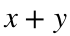.
• Subtract two numbers. That is, if x and y are numbers (not vectors), calculate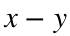.
• Multiply two numbers. That is, if x and y are numbers (not vectors), calculate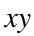.
• Divide two numbers. That is, if x and y are numbers (not vectors), calculate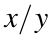.
• Multiply two numbers and add them to another. That is, if x, y and z are numbers (not vectors), calculate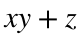.
The first four are probably a single instruction on every computer you have ever used. The fifth one (called a fused multiply add instruction) is common on modern processors, but takes two instructions on older computers.
(Side note: Many processors can also perform several of these operations at once. For instance, most modern laptops can add two pairs of (double precision) numbers together in the same amount of time they could add just one pair. MATLAB may or may not take advantage of this fact, depending on how you write your code, and it is well beyond the scope of this class. In any case, it won't affect our final estimates.)
When the numbers involved (x, y and z) are decimals, we call these instructions floating point operations, or flops. Our goal is to estimate how many flops it takes to solve an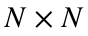system, then get an estimate of how long a flop takes to execute, then combine these to get an estimate of how much time it will take to solve the system.

### Speed of back/forward substitution

To begin, let's assume we are trying to solve ansystem of equations that is already in (upper/lower) triangular form. As we said in the last lecture, MATLAB uses back or forward substitution to solve these systems. We will illustrate these calculations with an upper triangular system, but it is easy to translate this argument to one for a lower triangular system as well.
In general, our problem will look like this:Solving for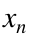takes only one flop: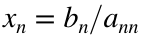can be calculated with a single division operation. Once we have, solving for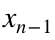takes two flops. We first do a fused multiply add to calculate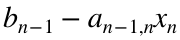, then a division to calculate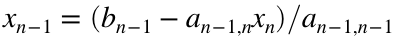. (It is easy to get confused by the first operation: It is written in a different order than our definition of a fused multiply add instruction, and there is a negative sign here. However, your processor calculates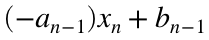. If you wrote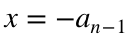,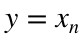and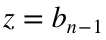, this would be the same as our original definition.) Continuing this pattern, each row takes one more flop until we get to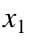, which takes N flops: One each to multiply the x-values we already know by their corresponding coefficients and subtract them to the right hand side, then one more to divide everything by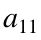.
This means that it takes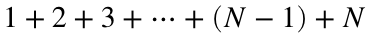flops to solve the whole system. It is easy to check that this adds up to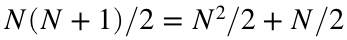total flops. We are only interested in a very rough estimate of the speed, so we will simplify this considerably. First, when N is very large (which is the only time when it really matters how fast our code is),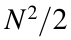is far bigger than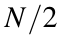. This means that theterm does not make a very big difference in our estimate, so we might as well just say it takesflops. Second, we don't really care about constant factors. There are several reasons why these factors are customarily ignored, but the most relevant to us is that the factor of 2 will make less difference in the final speed estimate than all the other approximations we are making about exactly what a flop is or how long it takes to execute. We will therefore just say that back/forward substitution takes approximately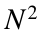flops.
We say that back/forward substitution is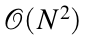(pronounced "order N squared" or "Big-oh of N squared"). In Big O notation, we ignore any constant factors and any terms with smaller powers, soand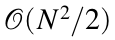and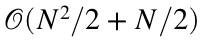are the same thing.
What good is this estimate? If we knew how long it took to execute a flop, we could then estimate how long it would take to solve a system with back/forward substitution. Let's say it takes t seconds to execute a flop. The total time for the whole algorithm is therefore approximately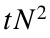. The key thing to notice here is that the t in our total time has a power of 1, while the N in our total time is raised to a power of 2. This means that if you get a computer that is ten times as fast (so t is ten times smaller), then your algorithm will take ten times less time to run. However, if you make your problem ten times as big (so N is ten times larger), then your algorithm will take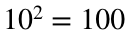times longer.
We will assume that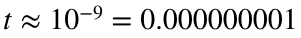seconds. There are a lot of complications that go into processor speed (in particular, not all operations take the same amount of time, and your processor spends a lot of its time doing other tasks besides solving linear systems, like printing to the screen or keeping the operating system running) but we can get decent order of magnitude estimates by using a small constant value like this.
This means that we could solve a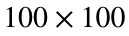system in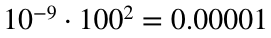seconds, while a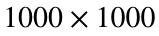system would take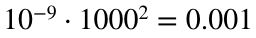seconds and a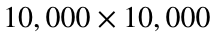system would take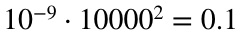seconds and a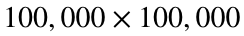system would take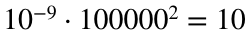seconds.
Ten seconds is not that slow, so this means that even quite large triangular systems can be solved in a reasonable time. (You probably can't even make amatrix on your personal computer, so practically speaking this is the upper limit on how long back substitution will take you.) You can verify these times yourself using the commands tic and toc. The tic command starts a timer and the toc command stops the timer and prints out how much time elapsed. For example, you could use the following code to make anupper triangular system and then time the backslash command with it.
N = 100;
% Make a random NxN matrix and zero out everything
% below the diagonal
A = rand(N);
for i = 1:N
for j = 1:i-1
A(i, j) = 0;
end
end
% Make a random vector for the right hand side
b = rand(N, 1);
% Time the solution
tic
A\b;
toc
Elapsed time is 0.003591 seconds.
The exact times you get will depend heavily on your exact computer and MATLAB setup. In particular, if you are using MATLAB online, you may or may not see the patterns we just discussed. This is because MATLAB online runs your code on a very fast mathworks server, and the computation time might be dominated by other factors (such as how many other people are running code on the same machine). I recommend you try this code with various values of N on a downloaded version of MATLAB (rather than online) with your computer plugged in (because laptops will usually throttle your processor if running on battery). This is also an interesting place to compare python and MATLAB if you are interested in using both.

### Speed of Gaussian elimination

Now let's do the same thing for a general (not triangular)system. Our standard approach is to eliminate all coefficients below the diagonal so that our system becomes upper triangular, then use back substitution to solve the resulting system. We already know that it takesflops to use back substitution, so we just need to check how many flops are needed for the elimination step.
For each coefficient, we have to multiply a row by some number and then add it to another row. That sounds like a fused multiply/add operation, but instead of operating on single numbers, we are operating on an entire row. This means that we have to use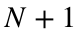flops to zero out a single coefficient - one multiply/add for each variable, plus one more for the right hand side. How many coefficients do we need to zero out? We need to eliminate everything below the diagonal, and there aretotal coefficients, but N of those are on the diagonal. This means that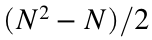are above the diagonal and anotherare below the diagonal, and so we have to zero outcoefficients. We also know that each coefficient takesflops to zero out. Therefore, the whole elimination process takes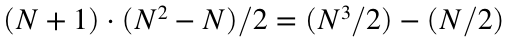flops. In addition, we just found out that the back substitution process takes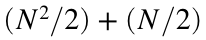additional flops, and so the entire process of Gaussian elmination takes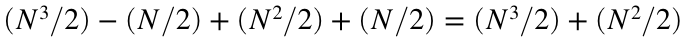flops.
Remember that in big O notation we ignore constant factors and terms with lower powers, so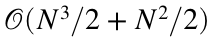is the same thing as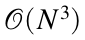. This means that we can just call the entire process of Gaussian elimination. (As above, this is a gross oversimplification, but when N is very large, the only important feature of this estimate is the biggest power of N, and so we get good qualitative estimates without worrying about the extra details.)
Just like with substitution, we can use this to estimate how long the backslash command will take. If we assume that an individual instruction takes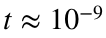seconds, then Gaussian elimination would take roughly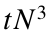seconds. As with substitution, these exact numbers are pretty close to meaningless; the key thing to notice here is the exponents and how the speed scales up as N changes. The t is only raised to the first power, while the N is raised to the third power. This means that if you get a computer that runs ten times as fast (so t is ten times smaller), the backslash command will run ten times quicker. However, if you make your problem ten times larger, then the backslash command will take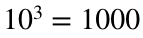times longer. For example, we could solve asystem in approximately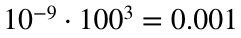second, while asystem would take roughly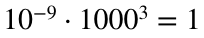seconds, and asystem would take approximately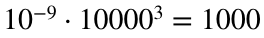seconds, or about 16 minutes. Asystem would take almost twelve days to solve. As you can see, the cubic power means that our run times will get out of hand very quickly.
As above, you can use the tic and toc commands to time MATLAB code yourself. We could time Gaussian elimination with code like this:
N = 100;
% Make a random NxN system
A = rand(N);
b = rand(N, 1);
% Time the solution
tic
A\b;
toc
Elapsed time is 152.670419 seconds.
Again, MATLAB online is not necessarily a good tool for timing code, because the elapsed time can sometimes depend on factors outside of your control more than the amount of work done by your code. It's also important to keep in mind that the exact times will vary a lot from run to run and computer to computer. What we are really interested in here is comparing different values of N and comparing different methods.